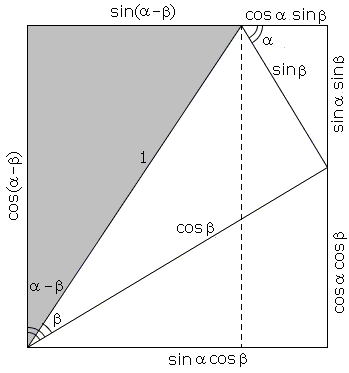# Addition and Subtraction Formulas for Sine and Cosine II

The simplest, truly "proofs without words" for the addition and subtraction formulas for sine and cosine have been published by R. Nelsen. As the other proofs relying on visual imagery, Nelsen's too are subject to natural argument (angle) limitations:

0 < α, β, α + β, < 90°, for addition, and
0 < α, β, α - β < 90°, for subtraction.

### Formulas for sin(α + β) and cos(α + β)sin(α + β) = sinα cos β + cos α sin β.
cos(α + β) = cosα cos β - sin α sin β.

### Formulas for sin(α - β) and cos(α - β)sin(α - β) = sinα cos β - cos α sin β.
cos(α - β) = cosα cos β + sin α sin β.

### References

1. R. B. Nelsen, Proofs Without Words II, MAA, 2000, p. 46### Trigonometry